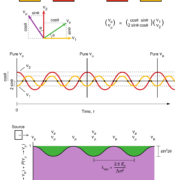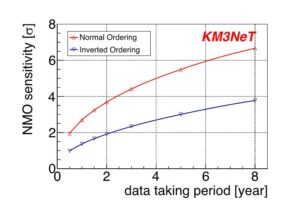# New publication: Neutrino Mass Ordering and Oscillation Parameters

05 May 2021 – The potential of KM3NeT to measure key properties of neutrinos – in March 2021, the KM3NeT Collaboration released a publication showing that  KM3NeT with its ORCA detector will be in an excellent position to study the phenomenon of neutrino oscillations!

#### Three neutrino flavours and oscillation

Neutrinos come in three species called flavours: the electron neutrino, the muon neutrino, and the tau neutrino. In the 1960’s, the first experiment was started to study the sun by measuring the flux of electron neutrinos that the solar nuclear processes copiously produce. The experiment revealed that the flux was inconsistent with the expectations! Many solutions were put forward to explain the discrepancy until a measurement of the flux of neutrinos of all three flavours was made and found compatible with the expectation. This key measurement meant that the expectations for the neutrino flux produced by the sun were correct and that the electron neutrinos were converted into other flavours while traveling to Earth. This phenomenon is called neutrino oscillation, subsequently detected also in other contexts. This phenomenon is only explained by quantum mechanics and requires that the neutrinos, initially thought massless, are actually massive!

The neutrinos with definite masses happen to be different from the neutrinos with definite flavours. In other words, a neutrino of a given flavour is an admixture of the neutrinos of definite mass as shown in the top part of fig:1. Because of the mass difference between the neutrino mass states, these states do not propagate at the same velocity. As a result, the neutrino admixture evolves during the propagation, as shown in the bottom part of fig:1. In other words, while propagating, the neutrino flavour changes.Figure 1: Top:the mass state admixtures corresponding to the flavour (so-called weak) states for 2 neutrinos. Middle: a muon neutrino is produced at t=0. As time goes, the neutrino mixture varies reaching periodically a pure muon neutrino state. The probability for the neutrino to be detected in each flavour is represented at the bottom. Reproduced from Slansky et al. Los Alamos Sci. 25 (1997) pp. 28-63.

#### Using atmospheric neutrinos

The KM3NeT Collaboration aims to study this oscillation phenomenon using neutrinos produced in the collisions of cosmic rays onto the atmosphere. Using these neutrinos, the KM3NeT Collaboration will be able to measure one of the key parameters ruling the neutrino admixture: the so-called θ23 mixing angle. We will also be able to measure the squared mass difference between two of the neutrino mass states – δm232 – and to tell which of the three mass states is the heaviest, i.e. determining the neutrino mass ordering as shown in fig:2. Finally, we will check if the standard three neutrino oscillation paradigm is valid by measuring the fraction of cosmic-ray induced neutrinos that have oscillated to the tau neutrino.Figure 2: Sensitivity to neutrino mass ordering as a function of data taking time for both normal (red upward pointing triangles) and inverted ordering (blue downward pointing triangles). See the paper for more details and the values of the oscillation parameters considered to obtain the result.

#### Unique potential

The publication relies on precise simulations to determine the sensitivity of the KM3NeT/ORCA detector to these parameters. The prospects show that the experiment has a unique ability to make these measurements and that world best results can be obtained in few years of data taking with the full detector.

The publication has been submitted to EPJ-C and is available as a pre-print as arXiv:2103.09885.